# Earth rotation

How fast is the place on the Earth's equator moving if the Earth's radius is 6378 km?

v =  1669.7565 km/h

### Step-by-step explanation: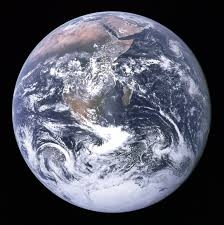Did you find an error or inaccuracy? Feel free to write us. Thank you!Tips to related online calculators
Do you want to convert length units?
Do you want to convert velocity (speed) units?
Do you want to convert time units like minutes to seconds?

#### You need to know the following knowledge to solve this word math problem:

We encourage you to watch this tutorial video on this math problem:

## Related math problems and questions:

• Pedestrian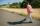Dana started at 10:00 from point A to point B. These points are distant 12 km. Determine how fast Daniel went when place B arrived at 11:54. Speed express in km/h.
• Motion against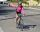From two places 36 km apart, a pedestrian and a cyclist will go straight opposite each other. How long will they meet if the speed of the pedestrian is 4 km/h and the cyclist 12 km/h?
• The cyclistThe cyclist drove from A to B, 60 km away. The first half drove at 30km/h, the second at 20km/h. How long did it take for him?
• The equator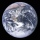Calculate the length of the equator. Calculate a radius of 6378 km. Round to the nearest thousand. - Procedure and calculation
• Earth and Sun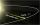Earth is 150 million km away from the Sun. 300,000 km of sunlight travels in 1 second. How long will the light come from the Sun to the Earth?
• Earth's diameter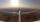The Earth's diameter on the equator is approximately 12750 km. How long does the Gripen fly over the Earth above the equator at 10 km if it is at an average speed of 1500 km / h?
• Rotation of the EarthCalculate the circumferential speed of the Earth's surface at a latitude of 61°​​. Consider a globe with a radius of 6378 km.The railroad runs parallel to the railway. The train travels at 36 km/h on track. In the opposite direction, the motorcycle rides at 90 km/h. The train passes in 3 seconds. How long is a train?
• Cyclist and carA cyclist who runs at a speed of 20km per hour will arrive at the finish in 4.5 hours. How fast does the car have to drive the same route in 2 hours?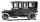Car started at 11:40 at speed 54 km/h from A and to B arrived at 12:20. Determine the distance A and B.
• DriverI drive at an average speed of 70km/h, I drive 10 thousand km. How long did it take me?
• Two vans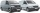At 8:30 a. M. , two vans leave at a parking area. They travel in opposite directions: one van travels at 48 km/h and the other at 62 km/h. At what time will the cars be 550 km apart?
• The EarthThe Earth's surface is 510,000,000 km2. Calculates the radius, equator length, and volume of the Earth, assuming the Earth has the shape of a sphere.
• Chase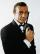Paul travel at 60 km/h. After 18 km ride found that he forgot an important book. His brother Thomas behind him carrying it. How fast must Thomas go to catch up with Paul in 31 minutes?
• Constant motionThe car has undergone constant motion 120 km in 1 hour 30 minutes. Determine its average speed. Determine how long the car drove in the first 20 minutes and 50 minutes from the start of the move.
• Average speed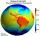What is the average speed you have to move the way around the world in 80 days? (Path along the equator, round to km/h).
• Thunderstorm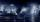The sound travels 1 km in about 3 seconds. How far is the storm if there is a time interval of 8 seconds between lightning and thunder?